Courses

# DC Shunt Generators Electrical Engineering (EE) Notes | EduRev

## Electrical Engineering (EE) : DC Shunt Generators Electrical Engineering (EE) Notes | EduRev

The document DC Shunt Generators Electrical Engineering (EE) Notes | EduRev is a part of the Electrical Engineering (EE) Course Electrical Machines.
All you need of Electrical Engineering (EE) at this link: Electrical Engineering (EE)

DC Shunt Generators
(Refer Slide Time: 00:22)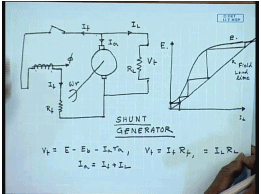In the last class, we have seen DC generator can be operated with a separately excited field, when this field excitation is supplied from a separate source. However, it is not necessary to excite the field winding from a separate source instead the generated by the generator itself can be used to provide the field current in which case the connection will look somewhat like this. Normally a resistance is included to control the field current, this is the terminal voltage V t and this is load current I L this is armature current I a this is field current I f.

In order to understand, how this circuit works? Let us first assume that the field is unexcited that is this which is open, with the field open, if the generator is run at rated voltage at rated speed initially the field current is 0 and the generated voltage will be equal to the residual voltage. And if field is current flows then the generated voltage will follow the O C C of the separately excited machine. This is the plot of E.

Now, initially the field current is 0 at this point the machine is run at it is rated speed and this which is closed. As soon as the switch is closed there is a current I f that will flow in the field circuit it is magnitude will be equal to the generated terminal voltage which is same as E here divided by the field resistance. So, this is the field resistance characteristics and this is field load line; however, we see that if this field current flows in such a direction that it hits the residual magnetism then the induced voltage in the armature will increase. Who which will then send more field current which will again increase the induced voltage and this process will continue till the voltage across the intersection point of the field load line with the O C C.

This process is called the self excitation of a d c shunt generator. Since the field winding is connected across the armature terminal, this is called a shunt connection or the generator is called a shunt generator. For this shunt generator then we can write V t equal to E minus E b which is the brush contract drop minus I a r a, also V t equal to I f R f equal to I L R L and I a equal to I f plus I L this is at the steady state equations of a DC shunt generator. Now, it has to be understood that this self excitation process requires certain conditions to be satisfied therefore, an appreciable voltage is generated across the terminal of the generator at no load.

(Refer Slide Time: 06:42)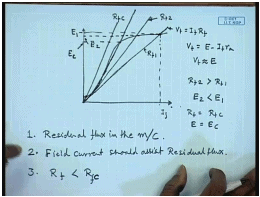Let us look at those conditions. If this is the O C C of the generator at rated speed and this is the field characteristics V t equal to I f R f at no load. V t equal to E minus I f r a we neglect the drop due to the small field resistance then V t is approximately equal to E that is the operating point at no load is determined by the intersection of the O C C with the field load line. So, this is the voltage at which the DC machine will excite to.

Clearly the voltage at which the DC machine will excite will depend not only on the O C C, but also on the field load line. For example, if the field winding resistance is increased, this is R f 1 this is the R f 2 then the voltage at which the terminal voltage of the machine at no load will change from E 1 to E 2. And if R f 2 is larger than R f 1, E 2 will be smaller than E 1. We can consider situation where, the field resistance is such that the field load line is tangent to the linear portion of the O C C in which case the excitation voltage will be E c. Clearly if the field resistance is anything more than f c then the induced voltage will drastically drop there will be almost no induced voltage at no load and in which case it will be called that the machine fails to excite.

Therefore we can see that self excitation does not occur at for all values of field circuit resistance there are in fact, three conditions for successful self excitation. First of all there must be residual flux in the machine, second field current; field coil connection should be such that field current assists residual flux. So, that as the field circuit is connected the flux keeps on increasing. Third the field circuit resistance must be less than the critical resistance of the field winding at the operating speed of the machine it is to be noted that the critical resistance, critical field resistance depends is not constant. In fact, it is depends on the machine operating speed which can be understood from the next diagram.

(Refer Slide Time: 11:59)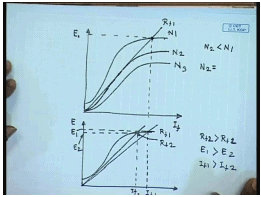Let us consider the shunt generator operating at a speed of N 1 when the O C C is given by this curve with the given field resistance. The operating no load voltage is determined by the intersection of the field load line with the O C C. Now we know that the O C C is proportional to the speed of the machine. So, at another speed N 2 it is entirely possible that with the same field resistance N 2. Now the O C C is tangent to the field load line evidently, if the speed of the machine is further reduced then with the same field current field resistance R f 1 the machine will fail to excite.

So, for every speed of operation of the DC shunt generator there is a critical field resistance below which the machine will fail to excite and also for every field resistance there is a critical operating speed below which the machine will fail to excite. Therefore, the third condition should be read as the field circuit total field circuit resistance must be less than the critical field circuit resistance at the given operating speed of the generator. If this three conditions are satisfied then a DC generator, DC shunt generator will go into self excitation and produce rated voltage at the terminal provided the field resistance is excited accordingly.

It should be understood also that, the terminal voltage at the generator at no load can be adjusted by controlling the field resistance. For example, at a given field resistance R f 1 the field current is I f 1 and the generated no load voltage is E 1, if the field circuit resistance is increased to a value of R f 2, the no load voltage as well as the field current required reduces to I f 2. If R f 1 is larger than R f 2, then R f 2 is larger than R f 1 then E 1 is larger than E 2 and I f 1 is larger than I f 2. Therefore, the no load terminal voltage of a shunt generator at any operating speed can be adjusted by adjusting the field circuit resistance. However as we have seen for separately excited DC generator it is more important to find out the output characteristics that is the external characteristics of a shunt generator.

(Refer Slide Time: 17:13)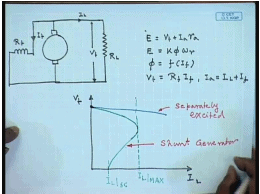For a shunt generator we know, E equal to V t plus I a r a, if we neglect the brush contract drop E equal to k phi omega r, phi equal to some non-linear function of I f, also V t equal to R f I f and I a equal to I L plus I f. If we plot the external characteristics of a DC shunt generator it is remarkably different from that of a separately excited d c generator. Even if the two machines are adjusted such that they produce the rated voltage at no load both as separately excited machine and a shunt machine if they are adjusted to produce the same no load voltage. For a separately excited machine the terminal voltage will drop, but for a shunt machine these voltage will drop much faster.

In fact, if we increase the load, the load current will increase up to a certain value after which it will decrease and even when the machine is short circuited, there will be only a finite load current I L S C flowing into the machine. So, this is the external characteristics of the same machine run as a separately excited generator. And this is the characteristics when it is excited as a shunt generator. In fact, these external characteristics can be obtained from the O C C of the machine graphically.

(Refer Slide Time: 21:56)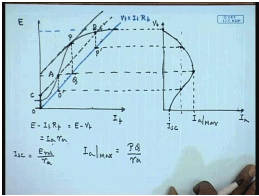The procedure is as follows. Let us first draw the O C C of the generator. This is the generator O C C, this is the field load line. So, at no load the terminal voltage is approximately given by this value. So, if we plot the external characteristics versus I a, if we draw V t then this point corresponds to the armature current of I f almost 0 very small.

At any other armature voltage at any other armature current, it should be appreciated that the difference between the O C C and the field load line given by E minus I f R f that is equal to E minus V t this is nothing but I a r a. So, this vertical distance between the O C C and the field load line is nothing but the armature drop therefore, we can find out the terminal voltage by the following procedure for any armature current, let us take the corresponding armature drop o c and draw a line parallel to the field load line. So, that the vertical difference between these two lines is always equal to o c equal to the I a r a.

We see that, this load line intersects the O C C at two points at A and at B. The vertical distance between the line section A O dashed and B P dashed are same as I a r a therefore, for the same armature current I a the terminal voltages will have two values. One corresponding to the point O dashed the other corresponding to the point P dashed. In similar manner other points can be drawn two points are of importance at terminal voltage equal to 0 the vertical I f equal to 0. So, the terminal voltage is 0 the induced voltage is given by the residual voltage.

Hence the short circuit current will be the residual voltage E residual divided by r a, this will be I S C. The maximum current that can flow in the armature of this DC machine can be obtained by drawing a line parallel to the field load line and touching the O C C at one point. So, the vertical distance of the point where this characteristics touches to the field load line, if the maximum I a, this is P this is Q.

So, I a max equal to P Q by r a and the corresponding terminal voltage is this point, this is and the external characteristics can be completed like this. This is the procedure for drawing the external characteristics of a DC shunt generator neglecting armature reaction. Please note that in this analysis we have neglected the armature reaction, we have not considered any drop due to armature reaction. Now if we want to incorporate armature reaction. Let us see how this procedure will modify.

(Refer Slide Time: 29:18)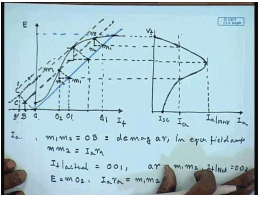Again we start by drawing the O C C of the generator, as well as the tilt load line corresponding to the field resistance to find out the no load terminal voltage. Since at no load there is no armature current there is no armature reaction so the terminal voltage is given by the intersection. At any other load current at any other armature current I a, we know that, the armature reaction then corresponds to a negative equivalent field amperes.

Let us say at any given armature current I a the corresponding equivalent armature reaction flux is O B and the armature resistance drop is given by B C. Now let us draw line parallel to the field load line through the point C again it intersects the O C C at two points m and n.

Now let us draw a line parallel to O C from m and n then we can draw the triangle O C B at points m and n, m, m 1, m 2, n, n 1, n 2; obviously, m1 m 2 equal to O B which is equal to defective demag armature reaction in equivalent field amperes. And m m 2 equal to I a r a therefore, for a actual field current corresponding to O O 1 and a actual armature current corresponding to I a, the effective filed current will be I f actual equal to O O 1 and a r ampere armature reaction demagnetizing field amperes is m 1 m 2 therefore, the net field ampere is I f net equal to O O 2. The corresponding induced voltage E equal to m O 2 and I a r a equal to m 1 m 2.

Therefore corresponding to a field current of I a there will be two terminal voltage given by O 1 m 1 and Q 1 n 1. So, these points can be now drawn on the external characteristics. For the short circuit condition the terminal voltage the field flux is very small, therefore there is almost no armature reaction effect therefore, the short circuit current will still be residual voltage by field resistance I S C.

For the maximum again through the maximum current we draw line parallel to field current then we extend the triangle here for the maximum armature current then the effective armature reactance, armature reaction demagnetizing amperes in equivalent field ampere will be O B dashed and the armature drop will be B dashed C dashed. Therefore, we draw a line parallel to O C dash from this point and the point, where it intersects with the field load line gives the value of the maximum, value of the terminal voltage at maximum armature current. And if we draw a triangle from here then I a r a equal to this length hence the I a can be found from there this will be I a max and the can thus be constructed this fashion.

Hence we see that in a shunt generator, the terminal voltage drops much faster than that of a separately excited generator the reasons being at in a separately excited generator. The armature current drop causes reduction in terminal voltage as well as armature reaction causes reduction in terminal voltage; however, the field current remains constant. In a shunt machine since the field winding is connected at the terminal of the generator as the terminal voltage reduces due to loading the field current also reduces hence the terminal voltage in a shunt generator drops much faster than that of a separately excited generator.

It is to be noted though. In fact, even under short circuit condition in only a finite amount of current flows in the armature. The armature current never exceeds this maximum limit; however, the normal operating region of a machine is much below this I max. Hence even though at short circuit the current may be smaller than the rated current of the machine transiently, if the terminals are shorted the armature current can exceeds the rated current of the machine and can damage the commutator segments. Now, again let us look at the previous problem.

(Refer Slide Time: 41:55)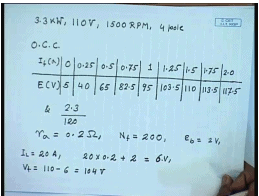The same machine in case it is operated as a shunt generator how the terminal voltage will vary. In this case, this was the given machine data that is 3.3 kilowatt 110 volt, 1500 R P M, 4 pole machine. The open circuit characteristics is given by this table, the armature resistance is 0.2 ohm, the number of field turns is 200 turns, the brush contract drop is 2 volts then we are also mentioned that the effective armature reaction ampere turns A R ampere turns equal to 1.5 times armature current I a. If this is machine is operated as a shunt generator let us try to find out the terminal voltage first by neglecting armature reaction and next time including armature reaction.

(Refer Slide Time: 43:25)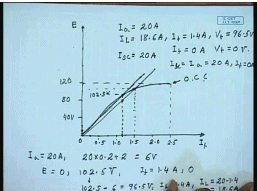The first step in solving this problem would be to draw the O C C of the generator. From the given data at no load the induced voltage is 5 volts, let us say this is 0. Let us say this is 0.5 amperes, this is 1 ampere, this is 1.5 ampere, this is 2 amperes and this is 2.5 amperes, let us this is 40 volts, this is 80 volts, this is 120 volts. This is the O C C, it is further said that, the field resistance is adjusted, so that at no load with rated field current of at no load the machine generate rated voltage. So, this is the field load line at the machine will generate 110 volt at no load and the corresponding field current will be 1.5 amperes.

So, if we neglect the armature reaction then in this diagram the length of this O B is 0. So, at any armature current we can draw a vertical line here; let us say for I a equal to 20 amperes, the total armature drop is 20 into 0.2 plus brush contract drop of 2 volts this gives you 6 volts. So, the corresponding 6 volts, we draw two lines a line parallel to the field resistance, this gives you two field currents E equal to approximately 0 and 102.5 volts. The corresponding field currents are I f equal to 1.4 ampere and 0 ampere, this is 102.5 volts, the corresponding terminal voltage V t corresponding to this will be 102.5 minus 6 equal to 96.5 volts, field current at that point is I f equal to 1.4 amperes therefore, the load current I L equal to 20 minus 1.4 equal to 18.6 ampere. So, one point on the corresponding to I a equal to 20 amperes is I L equal to 18.6 amperes, I f equal to 1.4 amperes and terminal voltage V t equal to 96.5 volts. The other point is I f equal to 0 ampere, V t equal to 0 volt and of course, I S C equal to 20 amperes. Since I f equal to 0, I L equal to I a equal to 20 ampere or I f equal to 0.

(Refer Slide Time: 51:48)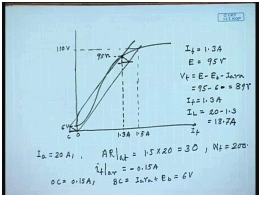Now, when we include armature reaction, the procedure gets slightly modified, but it is again starts with plotting the O C C and corresponding to 110 volt, the field current is 1.5 ampere, hence the load line, this is 6 volts. Now for again I a equal to 20 amperes, we know the A R a t equal to 1.5 into 20, that is 30, N f equal to 200 therefore, I f demagnetizing ampere turns in equivalent field amperes equal to minus 0.15 ampere.

So, let us draw a line showing minus 0.15 amperes and a line O C equal to 0.15 amperes and a line B C which is equal to I a r a plus E b is equal to 6 volts. And draw line parallel to the field load line, this intersects at V t equal to 95 volts and the corresponding field current is 1.3 amperes. So, the intersection point is at intersection point I f equal to 1.3 amperes, E equal to 95 volts. So, V t equal to E minus E b minus I a r a equal to 95 minus 6 equal to 89 volts, I f equal to 1.3 amperes and I L equal to 20 minus 1.3 equal to 18.7 amperes. This is how one can calculate the terminal voltage of a DC shunt generator.

Thank you.

Offer running on EduRev: Apply code STAYHOME200 to get INR 200 off on our premium plan EduRev Infinity!

61 docs|20 tests

,

,

,

,

,

,

,

,

,

,

,

,

,

,

,

,

,

,

,

,

,

;